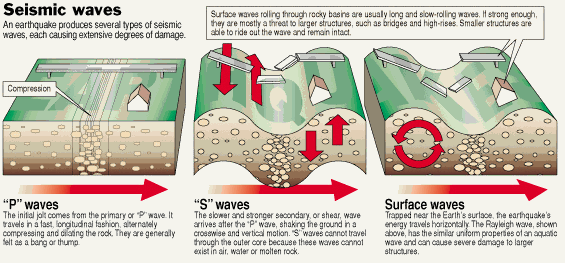Physics - Chapter 12Vibrations & Waves Lesson 62: Hooke’s Law and Simple Harmonic Motion Notes

NOTES

 The force provided by the spring is governed by Hooke’s Law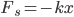k is spring constant, or sometimes called a force constant.The units are Newtons per meter.x is displacement from rest position of spring measured in meters. (m)x will be negative when the spring is stretchedx will be positive when the spring is compressed(-) because the spring is always providing a restoring force opposite the motion of the massThe (-) belongs to the x, not the k.Simple harmonic motion (SHM) occurs when the net force acting in the direction of motion follows Hooke’s Law.SHM will repeat a cycle of back and forth along same path forever.Also called periodic motion.Remember elastic potential energy can be found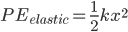The energy is only stored in a spring when it is either stretched or compressed.The potential energy in a spring is always positive.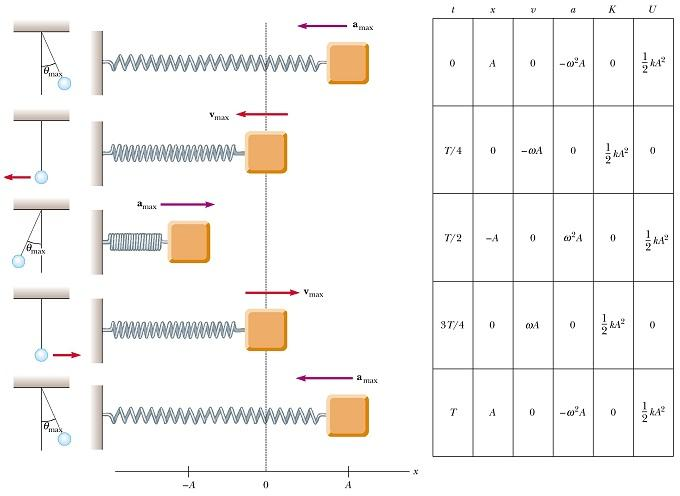EXAMPLE 1 – Solve the following using Hooke’s Law.

 A pinball machine uses a spring that is compressed 4.0 cm to launch a ball. If the spring constant is 13 N/m, what is the force on the ball at the moment the spring is released? A load of 45 N attached to a spring that is hanging vertically stretches the spring 0.14 m. What is the spring constant?

EXAMPLE 2 - A mass-spring system oscillates with an amplitude of 3.5 cm. The spring constant is 250 N/m and the
mass is 0.50
kg.

 Find the force on the mass at the instant the spring is released. Calculate the maximum acceleration of the mass-spring system. Find the maximum potential energy of the mass-spring system.

EXAMPLE 2 –Use the figure below to fill in the missing energy quantities.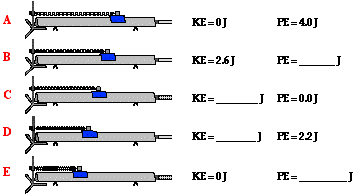Physics - Chapter 12 Lesson 63: Wave Types Notes

NOTES

 A transverse wave is a wave that its particles move perpendicular to the overall motion of the wave.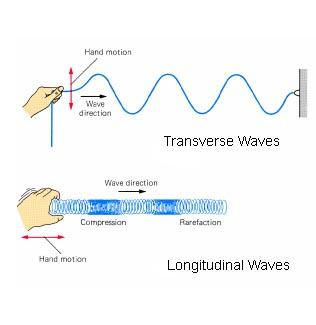A longitudinal wave is a wave in which its particles move in the same direction as the overall motion of the wave. Amplitude is the maximum distance object travels away from rest pointThe variable for amplitude is a capital AThe units for amplitude are metersPeriod is the time it takes to complete one full cycle of motionThe variable for period is a capital TThe units for the measuring the period are in seconds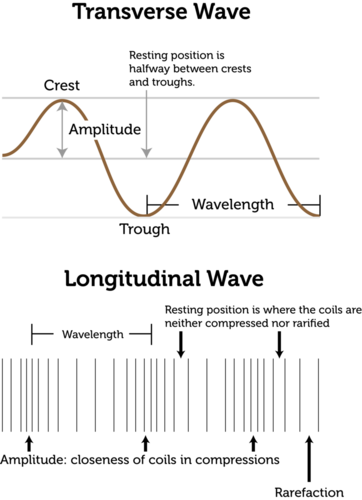Frequency is the number of cycles per unit of timeSaid another way as the number of waves past a given a point in one secondThe variable for frequency is the lower case Latin letterThe SI units for frequency is hertz (Hz)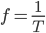Frequency is the inverse of the period                                The wavelength of a wave is the distance between two successive points on the wave.Typically measured from crest-to-crest.The variable used to represent wavelength is the lowercase Greek letter lambda, λ.Wavelength should be measured in meters.

EXAMPLE 1 – Use the figure at right to answer the following.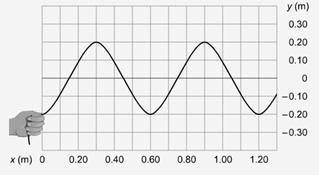Find the amplitude of the wave. Measure the wavelength. If the hand that generated the wave moved up and down two times in 8.0 s, what is the period of the wave? What is the frequency of the wave? What is the speed of the wave?

HOMEWORK -

 Physics - Chapter 12 Lesson 64: Pendulums Notes

NOTES

 A pendulum also exhibits simple harmonic motion under certain conditions.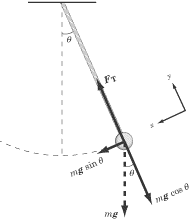The restoring force to maintain simple harmonic motion acts tangential to the path of the swing.A pendulum exhibiting simple harmonic motion will have a period that depends on:length of the pendulum, L longer the pendulum, longer the arc (larger amplitude)acceleration due to gravity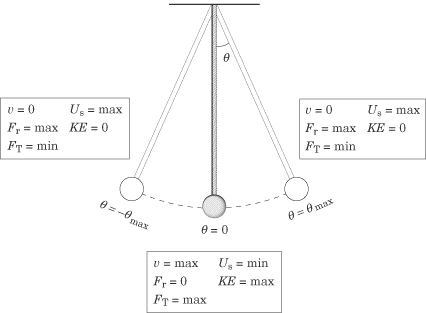The faster gravity can pull the pendulum, the shorter the time it takes to complete its cycle (period)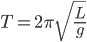To find the frequency of the wave, find the period and takes its inverse, or use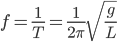Notice, the period of a pendulum does not depend on:mass of the pendulum bob, oramplitude of the swing

EXAMPLE 1 – Use the figure at right to answer the following.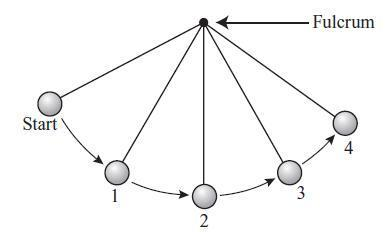At which location will the pendulum experience the greatest amount of gravitational potential energy? At which location will the pendulum experience the greatest amount of kinetic energy? At which location will the pendulum experience the greatest magnitude of force? At which location will the pendulum experience the greatest magnitude of acceleration? At which location will the pendulum experience the greatest magnitude of velocity?

EXAMPLE 3 – Solve the following.

 Calculate the period and frequency of a 3.500 m long pendulum swinging in Grant Park in Chicago, IL where the acceleration due to gravity is 9.803. A trapeze artist swings in simple harmonic motion with a period of 3.8 s. Calculate the length of the cables supporting the trapeze artist. What is the free-fall acceleration in a location where the period of a 0.850 m long pendulum is 1.86 s?

HOMEWORK -

 Physics - Chapter 12 Lesson 65: Mass-Spring System in Simple Harmonic Motion Notes

NOTES

 The period of a mass oscillating in simple harmonic motion depends onmass of the spring system, mthe force the spring can provide is set. So, F = ma tells us that acceleration will be less and kinematics says that time would be more!spring constant, kstiffer the spring, the less time it takes to accelerateIt only depends on these two things because gravity remains constant and those are the only two values that could vary in the system.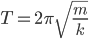Again, the frequency can be found by calculating the inverse of the period found in the equation above, or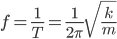Notice, the period of a mass-spring system does not depend on:acceleration due to gravity, oramplitude of the spring

EXAMPLE 1 – A spring constant of 30.0 N/m is attached to different masses, and the system is set in motion. Find the period and frequency of vibration for masses of the following magnitudes.

 2.3 kg 19 kg 15 g

HOMEWORK -

 Physics - Chapter 12 Lesson 66: Wave Speed and Interference Notes

LEARNING TARGET JOURNAL

 A wave pulse is sent down a rope that is attached to a pole in such a way that the end of the rope is free to slide relative to the pole.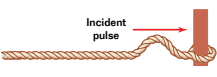Identify where the end of the rope will be when the incident pulse reaches the pole.Draw the reflected wave.A wave pulse is sent down a rope that is fixed to the pole.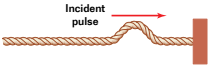Identify where the end of the rope will be when the incident pulse reaches the pole.Draw the reflected wave.

NOTES

 The speed of a wave front is the product of wavelength and frequency        the velocity (v) is measured in meters per second because𝜆λ is the wavelength measured in meters, and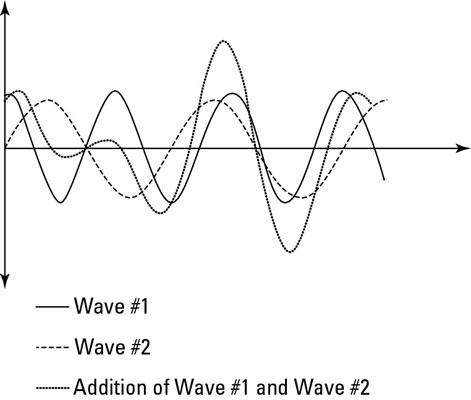ƒ is the frequency measured in hertzwhich is a per second measurementIf given the period of the wave, you must find the frequency as the inverse of the period before finding the speed of the wave.The Superposition Principle applies to when two or more waves traveling through the same medium try occupy the same place, the resultant wave is found by adding together the individual wave displacements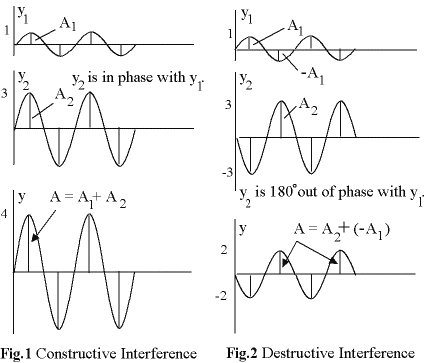Keep track of whether the wave displacement is positive (above the equilibrium position) or negative (below the equilibrium position). Constructive interference occurs when two waves meet that are in phase. Waves that are in phase have crests and valleys that line up exactly.This type will make a wave of larger amplitudeDestructive interference occurs when two waves meet out of phase.This will typically make a wave of smaller amplitudeComplete destructive interference will occur between waves of equal amplitude that are 180o out of phase.This would mean that a crest of one wave would interfere with the trough of the other wave.The result is that the waves will cancel each other out.

EXAMPLE 1 – Two wave pulses are sent towards each other in the same medium as illustrated in the following problems. Identify the type of interference and draw the resultant wave during interference.Type of Interference: Resultant Wave: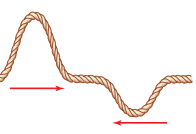Type of Interference: Resultant Wave: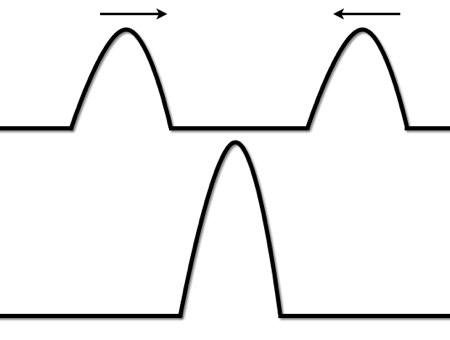Type of Interference: Resultant Wave: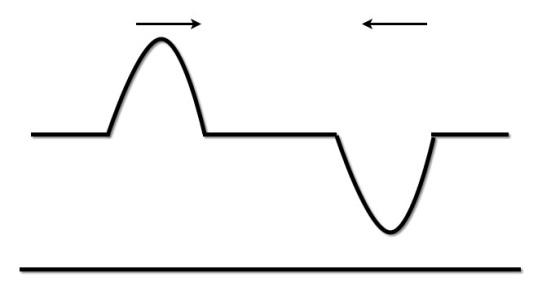Type of Interference: Resultant Wave:

EXAMPLE 2 – Which waveform of the three shown is the resultant wave? Explain how you identified the resultant wave.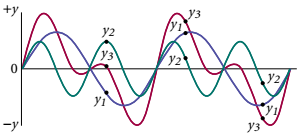EXAMPLE 3 – Solve the following.

 A tuning fork produces a sound with a frequency of 256 Hz and a wavelength in air of 1.35 m. What value does this give for the speed of sound in air? You dip your finger into a pan of water twice each second, producing waves with crests that are separated by 0.15 m. Determine the speed of these surface waves.

HOMEWORK -

 Physics - Chapter 12 Lesson X: Surface Waves & Pressure Waves Notes

NOTES

 A surface wave is a mechanical wave that travels along the surface, or boundary of two mediums.Surface waves in fluid act astransverse waves near the top of the fluid, andlongitudinal waves as you go deeper into the fluid.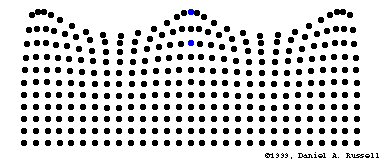A seismic wave is the type of wave associated with an earthquake.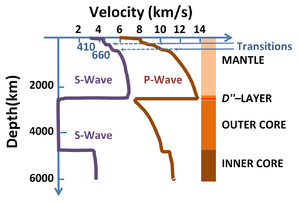Seismic waves actually come as two typesP-waves, or primary waves, travel as longitudinal compression waves.P-waves can travel the fastest, upwards of 14 km/s. S-waves, or secondary waves, follow p-waves and travel as transverse waves through loose portions of the Earth’s crust.As s-waves move closer to the surface, they begin to resemble surface waves.